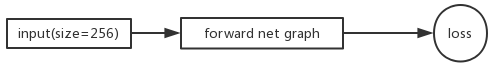# Why do we need to set the gradients manually to zero in pytorch?

@albanD: Which option is same as iter_size in caffe that very popular in Deeplab? Thanks

You need to change the inner check from:

``````if (i+1)%10 == 0:
``````

to

``````if (i+1)%iter_size == 0:
``````

Thanks but I meant option1, option2 or option3 in your answer will reproduce close performance with iter_size option in caffe?

All three answer compute the exact same gradients so they will be the same as using caffe with iter_size and a batch_size in caffe of `batch_size / iter_size` the same way in example 2 and 3 the batch size is reduced compared to example 1.

2 Likes

Hello, as u described above

``````Indeed, in the second case you will use much more memory. Indeed, for the 64 iterations, you will create a single graph that just keep growing, and so you will use more and more memory.
``````

Why is the size of the size-64 computation graph keep growing? Should not it be const size as the computation and the input size keep const?

1 Like

Pytorch does not freeze the graph until a `backward` call (or variables out of scope
https://discuss.pytorch.org/t/how-to-free-graph-manually/9255/2)
In the second case, so every operation like `total_loss = total_loss + loss` add new nodes to the graph.
So in every iteration, a subgraph with the same structure (if python logic is the same) but different values is add to the graph.
The graph is freed every 64 iterations on the call to `total_loss.backward()`

1 Like

I have another question:
if we have dataset of size 256

1. use all of them once to get the loss then backward
2. use 4 size-64 batch then sum up the loss to get the total one, after that do the backward

Which computation graph will be bigger?

Both computation graphs should be of same size (it does not matter if you forward one large or several smaller batches), since the intermediate values (which will be stored for backward computation, and which are the really memory consuming part of a graph) will be of the same effective size (maybe minor difference due to tensor management overhead when using multiple tensors instead of one large tensor, but I’d say this is negligible).

As we do forward computation, we get the computation graph for backward, and when we call `.backward()` , we get the grad and free the graph, am i right?

If you don’t specify other behavior (`retain_graph=True` or something like that) you are right.

As @justusschock said, the memory consumption is the same if neglecting overhead, but the graph structures are different. Illustrations are more clear:AFAIK, graphs cannot be explicitly manipulated. Only indirect ways like `backward` and name scoping are available.

3 Likes

Thank you!
But why you mention

graphs cannot be explicitly manipulated

what are are explicitly manipulation or what do you imply?

Sorry for confusing. I just wanna say, the computation graph is created and freed automatically, behind the operations.
Understanding what happend to graph is helpful.

Maybe it’s a good idea to add a graph semantic section in the documentation, elaborating various cases involving.

Do I only need to divide by `iter_size` if the loss function takes the average ?
Let’s say if I’m doing sum of squared errors, should I call backward() without dividing `loss` by `iter_size`?

Also, do I also need to be worried about batch normalization in this case if I don’t divide by `iter_size`?
Thanks!

Do you mean we should not zero out gradients for RNN? I thought the cell state would be doing most of the work of remembering the information.

This looks like the main reason why the design decision is made not to remove the gradients.
What I was trying to understand isn’t it the best time to remove the gradients after the optimizer step.

The idea is we don’t need to track when a certain functions ends, we just track the optimizer.
However, I am uncertain if this logic fits all the use cases, such as GANs, but I would like to hear the opinions.

I actually think zeroing gradients can be a design decision (with default to zero them).

hi alban…
I am doing the grad accumulation using this way… could you please let me know if there is any problem here

``````total_loss=0.
learn.model.train()
for i,(xb,yb) in enumerate(learn.data.train_dl):
#print('i',i)

loss = learn.loss_func(learn.model(xb), yb)
if (i+1) %2==0: # doing grad accumulation
loss=total_loss*0.9+loss*0.1 # some loss smoothning
#print('i',i)
loss.backward()
learn.opt.step()
total_loss=0.
else:
total_loss+= loss # accumulate the loss
``````
1 Like

Hi,

This looks good to me !

Hi,

It depends a lot on your usecase. sometimes you want to keep some gradients longer (to compute some statistics?).
But I agree that this is just a design decision. Unfortunately the original decision that was made was not to zero them and I don’t think we can change it now (for backward compatibility reasons).

1 Like

what if we don’t have an optimizer and just the model?